The Structure of the Atom

·      All atoms are made up of protons, neutrons and electrons

·      The protons and neutrons and found in the central nucleus and are collectively called nucleons

·      Electrons orbit the nucleus

·      The diameter of an atom is ~10-10m, a nucleus ~10-14m and a nucleon ~10-15m

 Proton Neutron Electron Charge + 1.60 x 10-19C 0 C -1.60 x 10-19C Relative Charge +1 0 -1 Mass 1.67 x 10-27kg 1.67 x 10-27kg 9.11 x 10-31kg Relative Mass +1 +1 0.0005

·      Atoms contain equal numbers of protons and electrons making them electrically neutral

·      Atoms with more or less electrons than protons are called ions and are charged.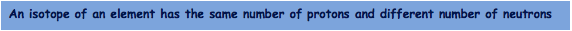Elements are defined by the number of protons they contain

A Z X Notation

·      All atomic nuclei are represented using this notation: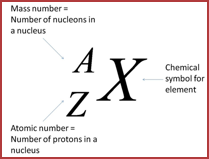·      The number of neutrons is found by: A-Z

E.g.  14 6 C

This is carbon and has 14 nucleons, of which 6 are protons. That means there are 14 _ 6 = 8 neutrons

Another isotope of carbon could be represented by 12 6 C. This would have 6 protons and 6 neutrons.

Specific Charge

·This is the ratio of a particles, atom or ions  charge (in C) to its mass (in kg)

·      It is important historically, as the charge to mass ratio of the electron was discovered before the individual values of mass and charge. It is important now in machines such as the mass spectrometer where charged particles are deflected by electric and magnetic fields. The amount of deflection is proportional to the particles specific charge.

·      Calculations:

-   For a particle (e.g. an electron), look up the charge and mass from the data sheet and divide.

e.g. an electron   charge/mass = -1.60 x 10-19/ 9.11 x 10-31 = -1.76 x 1011Ckg-1

-   For an atomic nucleus, add together the positive charges of all the protons and divide by the total mass of nucleons

e.g  14 6C nucleus  charge/mass = 6 x -1.60 x 10-19 / 14 x 1.67 x 10-27 = +4.11 x 107Ckg-1

-   For an ion, multiply the charge of the ion by a single unit of charge (the value e on the data sheet, 1.60 x 10-19C) and divide by the total mass of the nucleons plus the electrons

e.g. 52 24Cr2+ ion charge/mass = 2 x 1.60 x 10-19 / (52 x 1.67 x 10-27) + (22 x 9.11 x 10-31)

= +3.68 x 106 Ckg-1

·      An electron has the highest specific charge of any particle

Neutrinos

·      A neutrino is a fundamental particle that has no charge and (almost) no massThey do have energy and momentum

·      The symbol used in equations to denote  neutrinos and anti-neutrinos are:

The Strong Nuclear Force

·      There are four fundamental forces of nature

 Force Relative Stength Range (m) Description Strong Nuclear 1 10-15 Force between hadrons (particles made of quarks) Electromagnetic 10-2 ∞ Force between charges Weak Nuclear 10-6 10-18 Force between hadrons and leptons (responsible for β radioactive decay) Gravity 10-38 ∞ Force between masses

1 x 10-15m = 1 femtometer = 1fm

··Atomic nuclei are made of +ve protons and neutral neutrons. The protons therefore repel due to the electromagnetic force. They are only held together because they are made from quarks and therefore the strong nuclear force acts. It is much stronger than the electromagnetic force.

·      The strong nuclear force is so short range, its attraction can only be between adjacent nucleons

·      Think of the force like a spring linking nucleons, the harder you try to pull them apart, the more the spring reacts to hold them together until they are separated by too much  and the spring snaps. The particles go out of reach of the attractive nature of the strong nuclear force and repel away from each other by the electromagnetic force.

·      Some nuclei are unstable. We call these radioactive. Their nuclei decay (or change) to become more stable by emitting energy in the form of particles or radiation:

-   Alpha (α)

This is a particle made of 2 protons and 2 neutrons (the same as a Helium nucleus)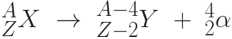-   Beta Minus (β-)

This is a fast moving electron made when a neutron changes into a proton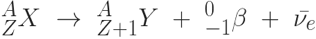-   Beta Plus (β+)

This is an anti-electron or positron emitted when a proton changes into a neutron-   Electron Capture

This is when an inner shell electron is captured by a proton in the nucleus, changing it into a neutron.-   Gamma (γ)

When protons and neutrons are left in an exited state by one of the other forms of radioactive decay, they may fall back to their lowest energy state by releasing a "packet" of energy in the form of a gamma ray. This is a pure emission of electromagnetic energy and does not affect the composition of the nucleus.The Photon Model of Light

·      Light is an electromagnetic wave that travels through a vacuum at a fixed velocity, c = 3 x 108ms-1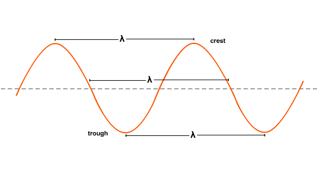·      The wave equation relates wavelength and frequency to velocity: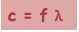c= wave speed (or velocity) = 3 x 108ms-1 in a vacuum

f = frequency (Hz)

λ = wavelength (m), typical values for visible light are 400 - 700 nm (= 400 - 700 x 10-9m)

·      Electromagnetic waves can also be thought to travel in small packets called photons . In this model, the energy of a photon is dependent on its frequency according to the equation: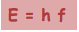E = energy of a photon (J)

h = Plancks constant = 6.63 x 10-34Js

f = frequency (Hz)

Converting Units of Energy·      The energies of photons are very small.  Physicists often use a different unit of energy for very small values called the Electron-Volt( eV)

·      1 eV is the energy needed to accelerate an electron through a potential difference of 1 Volt (in A2 Physics you will elarn about electric fields and the formula required to calculate this which is E = qV where q is the charge of the electron and V is the potential difference in volts. 1eV is therefore 1.60 x 10-19 x 1)

·      1 eV = 1.60 x 10-19J

·      1MeV = 1 x 106 eV

Anti-matter

·      Every particle has an anti-particle equivalent with the same mass but opposite charge (and other quantum properties)

E.g.

 Particle Symbol Mass (kg) Charge (C) Anti-particle Symbol Mass (kg) Charge (C) Electron e 9.11 x 10-31 -1.60 x 10-19 Anti-electron or positron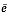9.11 x 10-31 +1.60 x 10-19 Proton p 1.67 x 10-27 +1.60 x 10-19 Anti-proton1.67 x 10-27 -1.60 x 10-19 Neutron n 1.67 x 10-27 0 Anti-neutron1.67 x 10-27 0 Neutrino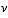~0 0 Anti-neutrino~0 0

·      If a matter particle meets its corresponding  anti-particle it will annihilate giving off a pair of high energy photons. This is an application of Einsteins famous E = mc2 equation. The energy of the photons given off are related to the mass of the particles annihilating.-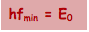The mimimum energy of each of the pair of photons given off is given by: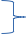h = Plancks constant = 6.63 x 10-34Js

fmin = frequency (Hz) of each of the pair of photons produced

E0 = Rest energy of one of the particles annihilating-Often the particle and anti-particle also have kinetic energy, Ek  in addition to their rest energy so:

·      The opposite process is called pair production. This is when a matter/anti-matter particle pair are spontaneously produced from a high energy photon in the presence of an atomic nucleus (which conserves momentum).  Again, E = mc2 means that the mass of the particles produced is related to the energy of the original photon.-The minimum energy photon needed to produce a pair is given by:

-If the photon has more energy than this, the remaining energy would be kinetic and the particles would move

To do:

1. Show that a photon must have a minimum energy of 1.02MeV to create an electron-positron pair.

2. Calculate the frequency of such a photon.

3. Calculate the minimum energy of each photon created when a proton and an anti-proton annihilate.

The Weak Nuclear Force and Feynman Diagrams

·      The weak nuclear force is an interaction that causes β decay

·      It affects all types of particles including hadrons and leptons

·      Like all four fundamental forces its action can be understood using exchange particles (or bosons) and Feynman diagrams.

 Force Exchange Particle (or boson) Electromagnetic Virtual Photons Weak Nuclear W+, W-  (and Z0 not required at AS level) Strong Nuclear Gluons (not required at AS level) Gravity Gravitons (postulated but not yet observed)

·      A virtual photon is one responsible for carrying the electromagnetic force – it is not observable as light in the usual way.

·      A Feynman diagram is a way of visualizing a particle interaction:

-   A Feynman diagram of a ball being thrown at a wall is shown below-   For the interaction between two colliding protons, it would look like:·      Notice that the axes are not drawn.

·      Feynman diagrams for the following weak or electromagnetic interactions need to be memorized:

-   Proton - Proton collision

-   Neutron-neutrino collision

-   Proton-antineutrino collision

-   Beta minus decay

-   Beta plus decay

-   Electron capture